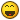xisto Community

## Recommended Posts

`Public Function GetPrimeNo (InNoWanted As Integer) As Long Dim lPrimes() As Long Dim lTrial As Long Dim lCount As Integer Dim lFound As Integer Dim lCounter As Integer ReDim lPrimes(InNoWanted)lTrial = 5 lCount = 3 lFound = 0 'Define the first three prime number ' > lPrimes(0) = 2 lPrimes(1) = 3 lPrimes(2) = 5 Do lTrial = lTrial + 2 lFound = 0 For lCounter = 0 To lCount - 1 lFound = (lTrial Mod lPrimes(lCounter)) = 0 If lFound Then Exit For End If lCounter = lCounter + 1 Next lCounter If lFound = 0 Then lPrimes(lCount) = lTrial lCount = lCount + 1 End If Loop While (lCount < InNoWanted) GetPrimeNo = lPrimes(InNoWanted - 1) End Function`

##### Share on other sites

That's great function... I'm really no good at math, and I can emember that on one or two occasions I needed such a function, to find a prime number... I'll keep this in my code base, for future reference... Great work##### Share on other sites

Are you sure that this works as I checked it at least I scanned it and it seems like it will return an error because of for loop. I am quite new to the visual basic but I am still learning that is in the process of learning. Maybe I am just wrong when I have more time I'll check it.

##### Share on other sites

I am reviewing your function once and you have a couple mistakes so far. I've been using VB for about 11 years now, and I will point them out to you, and post a bug free function.

You didn't post any information about how your function works. From what I can gather, the argument InNoWanted, will return the nth prime number, where n is the value of InNoWanted. For example, your function should produce the following results.

--------------------------------------
| InNoWanted | Value Returned |
--------------------------------------
| 1 | 2 |
--------------------------------------
| 2 | 3 |
--------------------------------------
| 3 | 5 |
--------------------------------------
| 4 | 7 |
--------------------------------------

The first error I have encountered is this that a value of zero will mess stuff up. You should add a check to make sure a value of zero is not entered:

`Public Function GetPrimeNo(InNoWanted As Integer) As LongDim lPrimes() As LongDim lTrial As LongDim lCount As IntegerDim lFound As IntegerDim lCounter As Integer	If InNoWanted = 0 Then	Exit Function	End If`

Nextly, it returns an error because you do not have enough elements in your array. You define 3 below this ReDim statement. Therefore you should add 3 to the amount of elements to create.

`ReDim lPrimes(InNoWanted + 3)`

You also commented out some code. You need that to have your program run correctly.
`'Define the first three prime numberlPrimes(0) = 2lPrimes(1) = 3lPrimes(2) = 5`

Other than that, you have no other programming mistakes in your code. Below is the fixed version:
`Public Function GetPrimeNo(InNoWanted As Integer) As LongDim lPrimes() As LongDim lTrial As LongDim lCount As IntegerDim lFound As IntegerDim lCounter As Integer	If InNoWanted = 0 Then	Exit Function	End IfReDim lPrimes(InNoWanted + 3)lTrial = 5lCount = 3lFound = 0'Define the first three prime numberlPrimes(0) = 2lPrimes(1) = 3lPrimes(2) = 5DolTrial = lTrial + 2lFound = 0For lCounter = 0 To lCount - 1lFound = (lTrial Mod lPrimes(lCounter)) = 0If lFound ThenExit ForEnd IflCounter = lCounter + 1Next lCounterIf lFound = 0 ThenlPrimes(lCount) = lTriallCount = lCount + 1End IfLoop While (lCount < InNoWanted)GetPrimeNo = lPrimes(InNoWanted - 1)End Function`

## Create an account

Register a new account